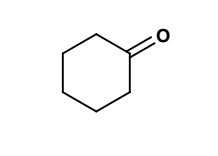# Problem: Splitting: For the structure below, indicate the splitting for each signal. Use these abbreviations for the splitting : s=singlet; d=doublet; t=triplet; q=quartet; etc. If there is complex splitting, indicate the total # of peaks and how the splitting is produced. e.g. d of t

###### FREE Expert Solution
98% (392 ratings)
###### Problem Details

Splitting: For the structure below, indicate the splitting for each signal. Use these abbreviations for the splitting : s=singlet; d=doublet; t=triplet; q=quartet; etc. If there is complex splitting, indicate the total # of peaks and how the splitting is produced. e.g. d of tFrequently Asked Questions

What scientific concept do you need to know in order to solve this problem?

Our tutors have indicated that to solve this problem you will need to apply the 1H NMR: Spin-Splitting Complex Tree Diagrams concept. You can view video lessons to learn 1H NMR: Spin-Splitting Complex Tree Diagrams. Or if you need more 1H NMR: Spin-Splitting Complex Tree Diagrams practice, you can also practice 1H NMR: Spin-Splitting Complex Tree Diagrams practice problems.

What is the difficulty of this problem?

Our tutors rated the difficulty ofSplitting: For the structure below, indicate the splitting f...as high difficulty.

How long does this problem take to solve?

Our expert Organic tutor, Chris took 9 minutes and 12 seconds to solve this problem. You can follow their steps in the video explanation above.